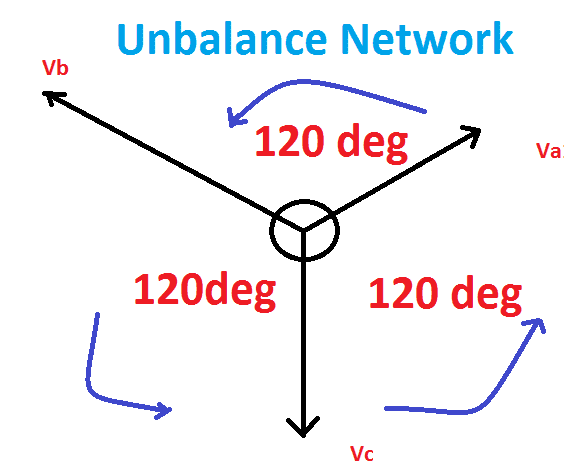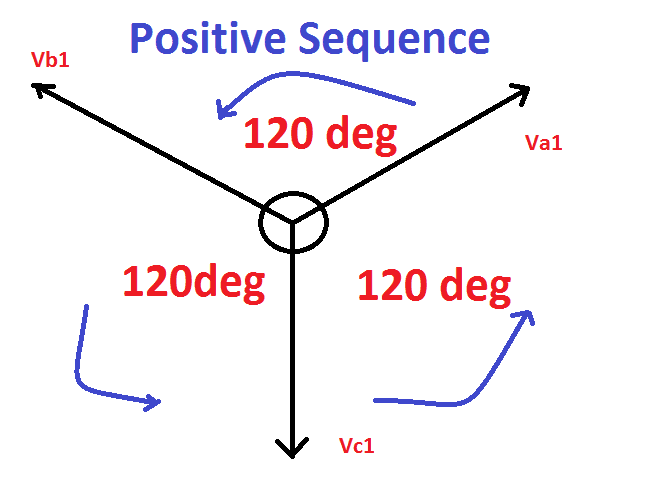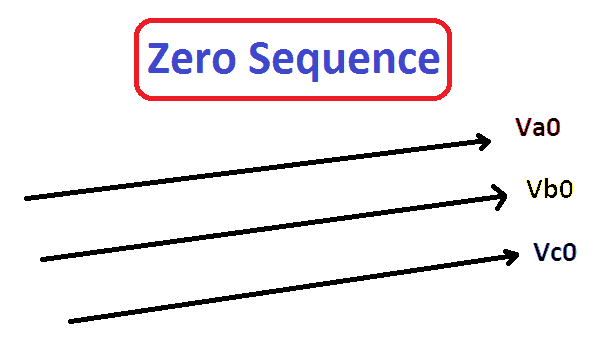# What is Symmetrical components Positive, Negative, Zero Sequence

## What is Symmetrical components:

Symmetrical components are nothing but a mathematical modeling which is used to analysis the unbalanced three phase power system both Normal and abnormal condition.In three phase system, The symmetrical components are referring three different unbalance phasors are

### Positive Sequence:

A balanced three-phase system with the same phase sequence as the original sequence. To say Positive Sequence components which should have three vectors of equal magnitude but displaced in phase from each other by an angle of 120° and have the phase sequence same as the original vectors. Phase sequence means the direction of rotation of vectors.### Negative sequence:

A balanced three-phase system with the opposite phase sequence as the original sequence which means the set of the three phasors are equal in magnitude, spaced 120° apart from each other and having the phase sequence opposite to that of the original phasors.

### Zero Sequence:

Three phasors that are equal in magnitude and phase.